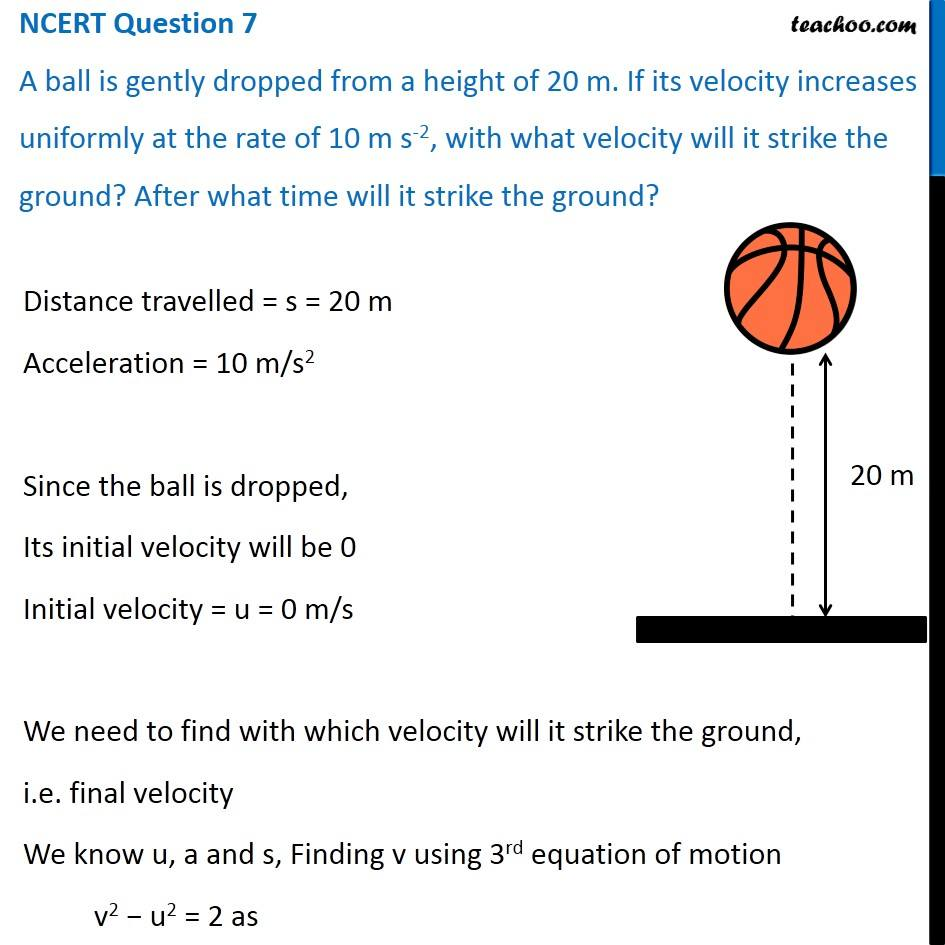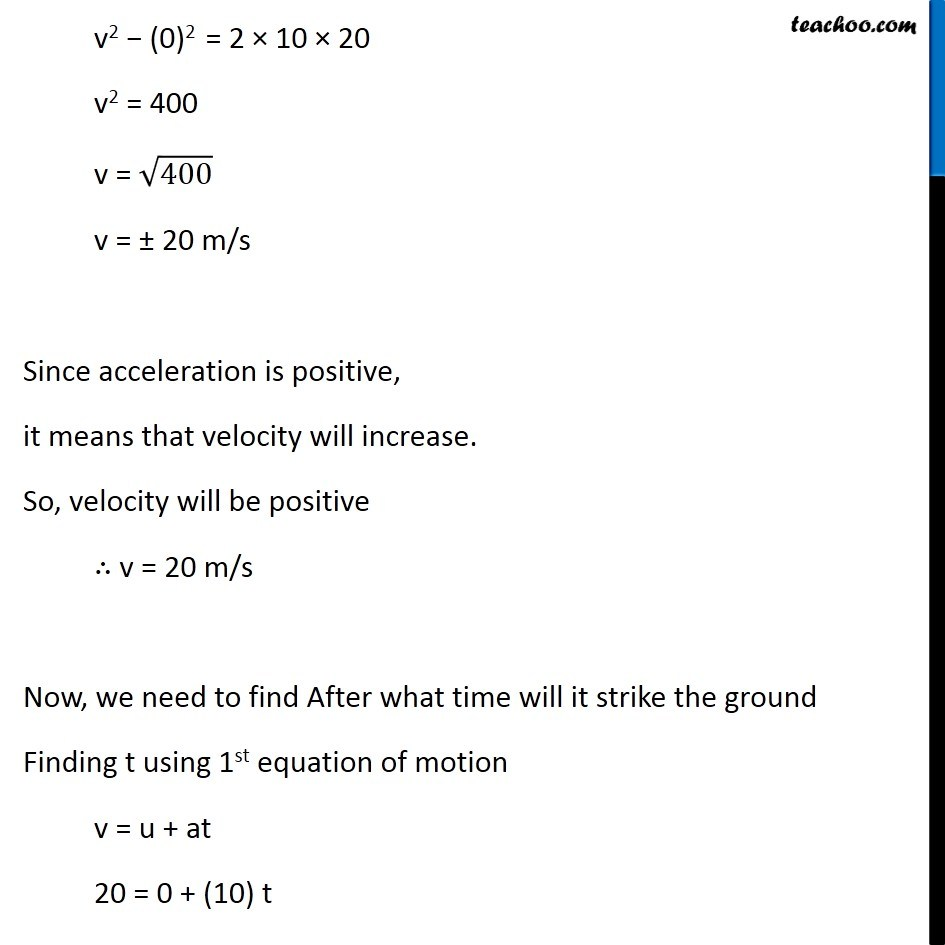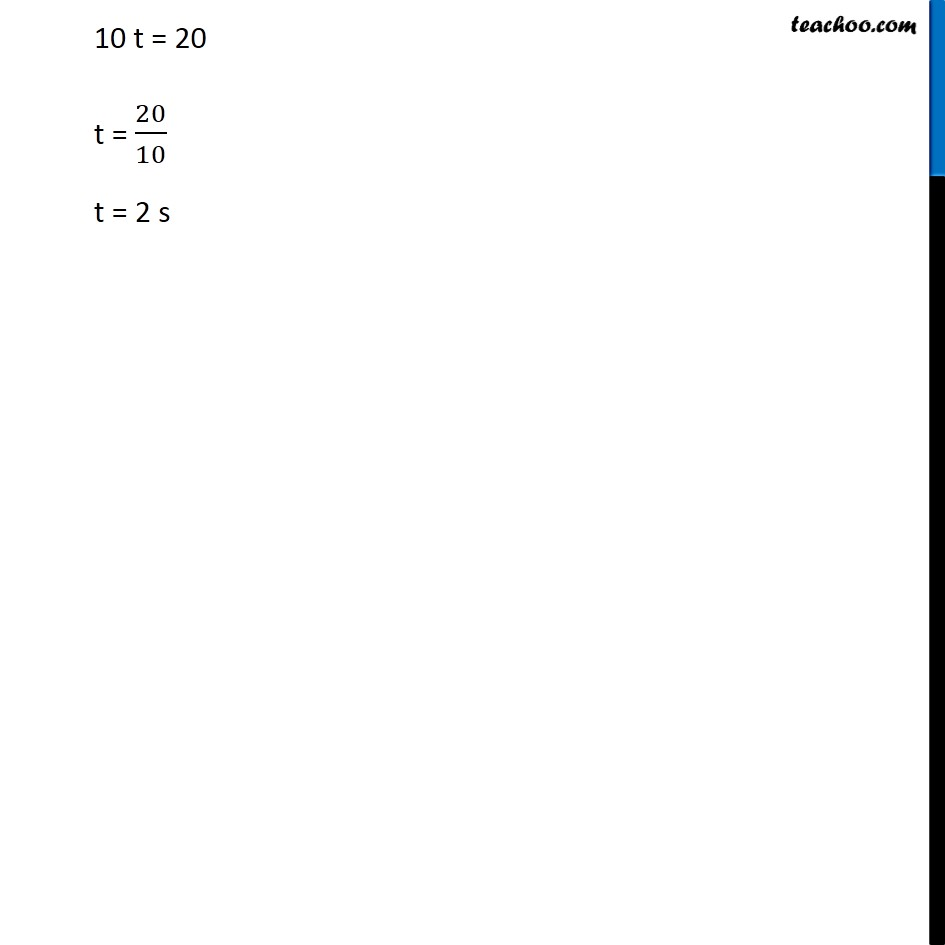NCERT Questions

Class 9
Chapter 8 Class 9 - MotionLearn in your speed, with individual attention - Teachoo Maths 1-on-1 Class

### Transcript

NCERT Question 7 A ball is gently dropped from a height of 20 m. If its velocity increases uniformly at the rate of 10 m s-2, with what velocity will it strike the ground? After what time will it strike the ground? Distance travelled = s = 20 m Acceleration = 10 m/s2 Since the ball is dropped, Its initial velocity will be 0 Initial velocity = u = 0 m/s We need to find with which velocity will it strike the ground, i.e. final velocity We know u, a and s, Finding v using 3rd equation of motion v2 − u2 = 2 as Distance travelled = s = 20 m Acceleration = 10 m/s2 Since the ball is dropped, Its initial velocity will be 0 Initial velocity = u = 0 m/s We need to find with which velocity will it strike the ground, i.e. final velocity We know u, a and s, Finding v using 3rd equation of motion v2 − u2 = 2 as 10 t = 20 t = 20/10 t = 2 s